﻿ The Effect of Gamma Irradiation on the Structural Properties of Porous Silicon

### The Effect of Gamma Irradiation on the Structural Properties of Porous Silicon

Ismail Khalaf Abbas, Laith Ahmed Najam, Abd UlKahliq AuobSulaimanOPEN ACCESSPEER-REVIEWED

## The Effect of Gamma Irradiation on the Structural Properties of Porous Silicon

Ismail Khalaf Abbas1, Laith Ahmed Najam1,, Abd UlKahliq AuobSulaiman1

1Department of Physics, College Of Science, Mosul University, Mosul, IRAQ

### Abstract

Porous silicon layers (PSi) were prepared from p-type silicon wafer by using electrochemical cell with etching time 20 min, current 30 mA and fixed electrolyte solution HF:C2H5OH (1:4). The effect of increase of γ-ray intensity (50Gy and 100Gy) on the structural properties of porous silicon has been studied using SEM, AFM, XRD and Raman spectrum. The SEM images before irradiation shows high density and randomly distributed of pores that cover all of the surface which have different size and spherical shape. After irradiation by 50Gy, the pores seems more obvious, discriminate and larger diameters. The initial elementary pores on the PSi surface decrease with the increasing of radiation intensity to 100Gy, as a result of formation of new pores with in the initial layer of Psi. The AFM images show that the roughness of the samples increase with irradiation. XRD spectrum before irradiation did not show clearly any featured peaks while the spectra after irradiation show the presence of different peaks but the most important distinctive was <111> peaks at ( 2θ = 28.12) which give indication that the structure is cubic. An extremely symmetric band shape were recognized from Raman spectra of the samples after and before irradiation.

### At a glance: Figures

1234
Prev Next

• Abbas, Ismail Khalaf, Laith Ahmed Najam, and Abd UlKahliq AuobSulaiman. "The Effect of Gamma Irradiation on the Structural Properties of Porous Silicon." International Journal of Physics 3.1 (2015): 1-7.
• Abbas, I. K. , Najam, L. A. , & AuobSulaiman, A. U. (2015). The Effect of Gamma Irradiation on the Structural Properties of Porous Silicon. International Journal of Physics, 3(1), 1-7.
• Abbas, Ismail Khalaf, Laith Ahmed Najam, and Abd UlKahliq AuobSulaiman. "The Effect of Gamma Irradiation on the Structural Properties of Porous Silicon." International Journal of Physics 3, no. 1 (2015): 1-7.

 Import into BibTeX Import into EndNote Import into RefMan Import into RefWorks

### 1. Introduction

Crystalline silicon is the most widely used semiconductor because of its physical and electrical property such as a small indirect band gap (1.12eV) and emits light weakly near the infrared region by phonon assistance .

Porous silicon was discovered in 1956 by Ulhir while performing electropolishing experiments on silicon wafers using an electrolyte containing hydrofluoric acid (HF), it can be considered as a silicon crystal having a network of voids . In the 1970s and 1980s, interest in PSi increased because of its high surface area was found to be useful as a model of the crystalline silicon surface in spectroscopy studies, As a consequence, it has become the favor material for sensing applications because of the low power consumption, low cost and its compatibility with silicon –based technologies .

The physical properties of porous silicon are fundamentally determined by the shape and diameter of pores, it has a wide area of potential applications such as waveguides, 1D photonic crystals, chemical and biological sensors .

In this study we were prepared porous silicon layer by using electrochemical cell with etching time of about 20min, current 30mA and electrolyte solution HF:C2H5OH (1:4). The SEM, AFM, XRD and Raman spectrum have been used to characterized the morphology and structural properties of the samples before and after irradiation by 50Gy and 100Gy gamma ray.

### 2. Experimental

P-type silicon wafer of <111> orientation was used as a substrate for the samples. The wafer was divided in a small pieces (1cm x 1cm). The wafer surface have been cleaned using a suitable solutions such as Isopropanol, Methanol, Hydrofloric acid and Aceton.

The samples were prepared using electrochemical cell as shown in Figure 1, the cell is made out of Teflon which is resistive against attack from the Hydrofloric acid electrolyte.

Figure 1. a schematic diagram of the circuit used in the samples preparation

The silicon wafer serves as the anode and it is sandwiched between the top and the bottom parts of the Teflon.

The cathode is a circular gold that is submerged in the Hydrofloric acid electrolyte, the cathode is held in place by the top part of the Teflon cell and an aluminum ring. The hydrofloric acid electrolyte is placed inside the top part of the Teflon cell as shown in Figure 2. Enough electrolyte must be present to supply the required fluorine ions and to cover the gold cathode. The top part of the Teflon cell has a circular window of area 1cm2, which exposes the silicon to hydrofloric acid and form the porous silicon. The electrochemical process is carried out under constant current 30mA and period of about 20min.

### 3. Results and Discussion

Porous silicon layers have been prepared from p-type silicon wafer using electrochemical cell. The process has been carried out of a constant current 30mA and a period of about 20 min. Samples surfaces were imaged before and after irradiation using scanning electron microscopy (SEM) and atomic force microscopy (AFM) in addition to X-ray diffraction (XRD) and Raman spectrum.

Figure 3 shows SEM images of porous silicon without irradiation in a different magnification (1000X, 5000X, 10000X, 20000X). We observed the emergence of high density numerous pores which randomly distributed on the surface of PSi, in order to increase the visibility of pores formed in silicon we have been enlarge magnification degree of samples images.

Figure 3. SEM images of porous silicon without irradiation in a different magnification degree) x1000, x5000, x10000, x20000)

After irradiation by 50Gy gamma ray, we note that the pores diameter increases since the radiation is destroy the thin walls between neighbor pores and cover all over the sample surface which clearly recognized at 20000X Figure 4.

Figure 4. SEM images of porous silicon after irradiation by 50Gy gamma ray in a different magnification degree (x1000, x5000, x10000, x20000)
Figure 5. SEM images of porous silicon after irradiation by 100Gy gamma ray in a different magnification degree) x1000, x5000, x10000, x20000)

Figure 5 shows SEM images of samples after irradiation by 100Gy gamma ray, we note that the pores possess medium volume as a result of pores diameter increase and this reduce the number of pores on the PSi surface causing low porosity. It is worth mentioning that the increase in irradiation power will decrease the porosity and this was deduced by comparing the dimensions of the pores irradiated by 50Gy and 100Gy gamma ray .

From Figure 6, the band gape of porous silicon was calculated for the samples before and after irradiation from photoluminescence spectrum, the band gap was determined for un-irradiated samples of about (2.02 eV) and decreases with irradiation power to about (1.96 eV) for 50 Gy and (1.77 eV) for 100 Gygamma ray .

Figure 6. the photoluminescence for porous silicon (a) before irradiation, (b) after 50 Gy gamma ray irradiation and (c) after 100 Gy gamma ray irradiation

The mean pores diameter in the PSi layer on the p-type silicon substrate was calculated form the following equation :(1)

E(eV): band gap that was calculated from photoluminescence spectrum.

Eg: band gap of the bulk silicon (eV)

h: Plank constant

m*h, m*e: electron and hole effective mass respectively.

m*e =0.19 m0 and m*h =0.16 m*h =0.16 m0 at room temperature.

The calculated pores diameter for PSi was (9.32 nm) for un- irradiated species, (9.65 nm) for the samples that was irradiated by 50 Gy and (10.97 nm) for the samples that was irradiated by 100 Gy gamma rays.

The roughness of the samples surfaces before and after irradiation was studied using AFM. Figure 7 shows that there is variation in the values of the surface roughness and whenever the amount of the gamma irradiation increased the surface roughness rate increase. Thus the surface roughness rate is directly proportional to the amount of irradiation, as well as we noted that the distribution of the pores silicon was random, irregular and the spaces (voids) covers all over the surface [4, 8].

Figure 7. AFM images of porous silicon surfaces (a) before irradiation, (b) after 50 Gy gamma ray irradiation and (c) after 100 Gy gamma ray irradiation

X-ray diffraction (XRD) technique was used to examine the crystalline structure of the p-type porous silicon layers. The (XRD) spectrum of the samples was analyzed before and after irradiation as shown in Figure 8.

Before irradiation, from the (XRD) spectrum shown in Figure 8-a we observe the existence of a distinctive cubic peak (111) at diffraction angle of about 2θ=28.12° in addition to another low intensity peaks such as (220), (311), (400), (331), (422) and (511) at diffraction angles 46.9°, 56.25°, 69.9°, 77.25°, 88.12° and 96.88° respectively.

After irradiation with 50Gy gamma ray, the relative intensity of distinctive<111> and other peaks were increased, this increase in the relative intensity of each peak refers to the formation of pores that are affected by the process of irradiation as in Figure 8-b.

Irradiation by 100Gy shown in Figure 8-c, we note that the increase in the amount of irradiation will produce a decrease in some peaks intensity and a rise in the others, this variation in the relative intensity of the peaks can be attributed to the irradiated porous silicon surfaces at 100Gy which reduces the number of pores and this is clear from the SEM images.

Figure 8. The X-rays spectrum of porous silicon (a) before irradiation, (b) after 50 Gy gamma ray irradiation and (c) after 100 Gy gamma ray irradiation

Figure 9 shows the (XRD) spectrum for porous silicon <111> before and after irradiation at a diffraction angles 25°-35°. The increase in the irradiation power produce an increase in the full width half maximum (FWHM) of distinctive peak <111>[1, 9, 10].

Figure 9. (XRD)spectrum for porous silicon (111) (a) before irradiation, (b) after 50 Gy gamma ray irradiation and (c) after 100 Gy gamma ray irradiation at a diffraction angles 25°-35°

Figure 10 shows Raman spectrum before and after irradiation for p-type porous silicon of <111> orientation, the band shape was extremely symmetric for all cases and the phonon frequency (phonon peak shift) appears at (515cm-1) which is used to determine crystal size as in the following equation :(2): Lattice constant = 0.543 Ao.

A= 52.3, γ=1.586

L: crystal volume = 2.12nm.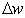optical phonon shift between the location of Raman band of PSi () and the location of bulk Si (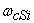=521 cm-1) as given in the following equation: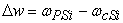(3)
Figure 10. show Raman spectrum (a) before irradiation, (b) after 50 Gy gamma ray irradiation and (c) after 100 Gy gamma ray irradiation

The crystal volume (D) was determined in another way using the following equations [11, 12, 13, 14]: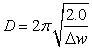(4)

D: crystal volume = 2.09 nmoptical phonon shift between the location of Raman band of PSi () and the location of bulk Si (=521 cm-1) as given in the following equation :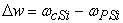### 4. Conclusions

•  Before irradiation process, a high density numerous pores emerged which distributed randomly on the surface of PSi. After irradiation by 50Gy gamma ray, the pores diameter increases and by increasing the irradiation the diameter increases more, the number of pores on the surface of PSi causing low porosity.

•  The band gap was determined for unirradiated samples of about (2.02 eV) and decreases with irradiation power to about (1.96 eV) for 50 Gy and (1.77 eV) for 100 Gy gamma ray.

•  The surface roughness rate is directly proportional to the amount of irradiation. The distribution of the pores silicon was random, irregular and the spaces (voids) covers all over the surface.

• The (XRD) spectrum before irradiation showed the existence of a distinctive cubic peak <111> in addition to another low intensity peaks. After irradiation with 50Gy and 100Gy gamma ray, the peaks intensities varied randomly.

•  From Raman spectrum before and after irradiation, the band shape was extremely symmetric for all cases.

### References

  Ma., Concepc, N., Arenas, Marina Vega, OmarMartnez and Oscar H. Salinas, (2011), “Nanocrystalline Porous Silicon: Structural, Optical, Electrical and Photovoltaic Properties crystalline properties and uses", prof. Sukumar, Bash (Ed), ISBN:251, intecech.In article  N., Naderi, M.R., Hashim, (2012), “Effect of Surface Morphology on Electrical Properties of Electrochemically-Etched Porous Silicon Photodetectors ", Int. J. Electrochem. Sci., 7, p.11512-11518.In article  KasraBehzad, Wan Mahmood Mat Yunus, ZainalAbidinTalib, AzmiZakaria and AfarinBahrami, (2013), "Effect of Preparation Parameters on Physical, Thermal and Optical Properties of n-type Porous Silicon ", Int. J. Electrochem. Sci., 7, p.8266-8275.In article  Hasan, H. H.,(2013), "study of characteristics of porous silicon by electrochemical etching ", Eng. and Tech. Journal, Vol.31, No.1.In article  N., Jeyakumaran, B., Natarajan, S., Ramamurthy and V., Vasu, (2007), "Structural and optical properties of n- type porous silicon– effect of etching time", IJNN, Vol.3, No.1.In article  Ma, S.Y., Zhang, B.R. and Qin, G.G., (1997), "Effects Of Y-Ray Irradiation On Photoluminescence Spectra From S & Rich Silicon Oxide", Materials Research Bulletin, Vol.32, No.10, p.1427-1433.In article CrossRef  Bisi, O., Ossicini, S., Pavesi, L., (2000), "porous silicon: a quantum sponge structure for silicon based optoelectronics, surface science", reports 38, p. 1-126.In article  Khaldun, A., Salman, Z., Hassan, Khalid Omar, (2012), “Effect of Silicon Porosity on Solar Cell Efficiency", Int. J. Electrochem. Sci., p.7 376-386.In article  Klug, H. P., (1954), "crystallite size and lattice strains, in X-ray diffraction procedures", Newyork:wiley – intersience: kluge H.P. and Alex and er. L.E., p. 656-657.In article  Mingyuan, Ge, JiepenRony, Xin Fang, Any Zhang yunhao Luand Choywu, Zhou, (2013), "Scalable preparation of porous silicon nanoparticles and their application for Lithium ion battery anodes", Nano Res., 6 (3), p. 174-181.In article CrossRef  Paillard, V., Puech, P., Laguna, M.A., Carkes, R.,Kohn, B. Huisken, F., (1999), "improved one phonon confinement model for an accurate size determination of silicon nanocrystal", J.Appl.Phys. Vol.86, No.4, p.1921-1924.In article CrossRef  Beeman, D., Tsur., Thorpe, MF, (1985), "structural information from the Raman spectrum of amorphous silicon", phys. Review, B., Vol.32, p.874-878.In article  Sui, Z., Leong, PP., Herman, IP., (1992), "raman analyses of light emitting porous silicon", Applied Phys. Lett., Vol.60, p.2086-2088.In article CrossRef  He, y., Yin, Cy., Cheng, G., Wang, L., Liu, X., Hu, Gy., (1994), "the structures and properties of nano sized crystalline silicon films", J. Appl., Phys., Vol.75, p.797-803.In article